# String Wave Solutions

A solution to the wave equation for an ideal string can take the form of a traveling wave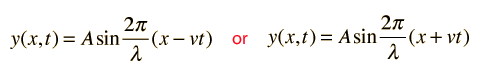Show

For a string of length L which is fixed at both ends, the solution can take the form of standing waves: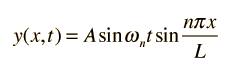Show

For different initial conditions on such a string, the standing wave solution can be expressed to an arbitrary degree of precision by a Fourier seriesCalculation of parameters Wave packet solution
Index

Wave concepts

 HyperPhysics***** Sound R Nave
Go Back

# Traveling Wave Solution for String

A useful solution to the wave equation for an ideal string is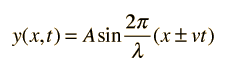Show wave parameters
 Show that -vt implies velocity in +x direction

It can be shown to be a solution to the one-dimensional wave equation by direct substitution: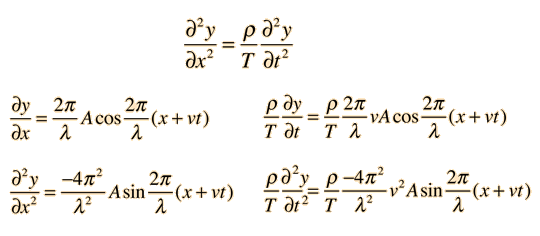Setting the final two expressions equal to each other and factoring out the common terms givesThese two expressions are equal for all values of x and t and therefore represent a valid solution if the wave velocity isWave velocity for a stretched string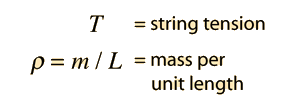Show details Solutions to wave equation
Index

Wave concepts

 HyperPhysics***** Sound R Nave
Go Back

# String Traveling Wave Velocity

 For a point of constant height moving to the right: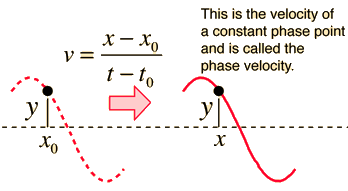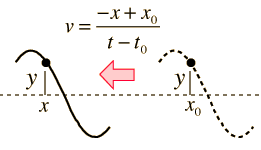For a point of constant height moving to the left: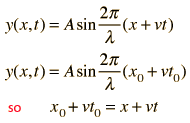From the traveling wave solution, the phase velocity for a string wave is given by:Index

Wave concepts

 HyperPhysics***** Sound R Nave
Go Back

# Traveling Wave Parameters

A traveling wave solution to the wave equation may be written in several different ways with different choices of related parameters. These include the basic periodic motion parameters amplitude, period and frequency.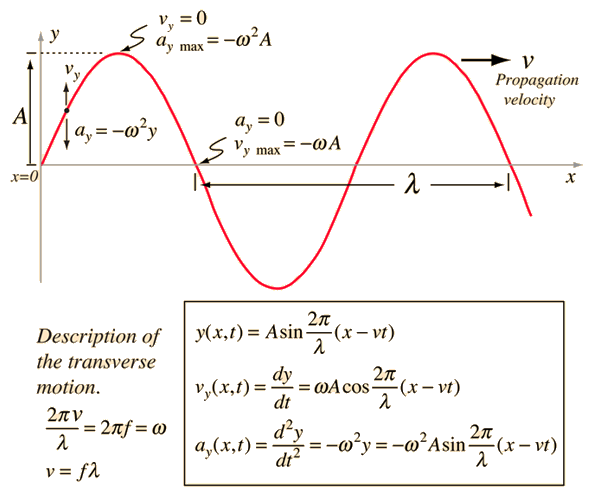Equivalent forms of wave solution: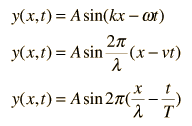Wave parameters:
 *Amplitude A *Period T = 1/f *Frequency f = 1/T *Propagation speed v *Wavelength λ *Wave number k = 2π/λ *Angular frequency ω = 2πf *Wave relationship v = fλ

The wave velocity is determined by the properties of the medium and is independent of the other parameters, but it can be determined from measurements of the frequency and wavelength. The following calculation allows you to specify any two of the quantities in the wave relationship v = fl and then initiate the calculation by clicking on the active text for the quantity you wish to calculate.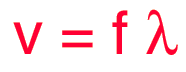## Wave velocity = frequency x wavelength

### Wavelength = m = x10^m = x10^ft. Frequency = Hz = x10^Hz Wave velocity = m/s =x10^m/s = x10^ft/s. Wave number k = m-1 =x10^m-1. Angular frequency ω = rad/s =x10^rad/s. If the wave has amplitude A = mand initial phase φ = degrees = radiansthen at x = m and time t = s the wave can be described by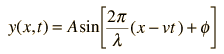= m with ymax=A= m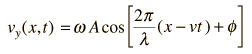= m/s with vy max = ωA = m/s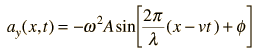= m/s2 with amax = -ω2A = m/s2

Note: The position, velocity and acceleration are recalulated only when you click on either velocity, frequency or wavelength in the active formula above.

 Complex number form
Index

Wave concepts

 HyperPhysics***** Sound R Nave
Go Back

# Plane Wave Expressions

A traveling wave which is confined to one plane in space and varies sinusoidally in both space and time can be expressed as combinations of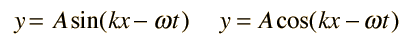It is sometimes convenient to use the complex form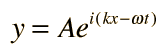which may be shown to be a combination of the above forms by the use of the Euler identity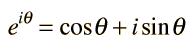In the case of classical waves, either the real or the imaginary part is chosen since the wave must be real, but for application to quantum mechanical wavefunctions such as that for a free particle, the complex form may be retained.

 Wave parameters String waves
Index

Wave concepts

 HyperPhysics***** Sound R Nave
Go Back